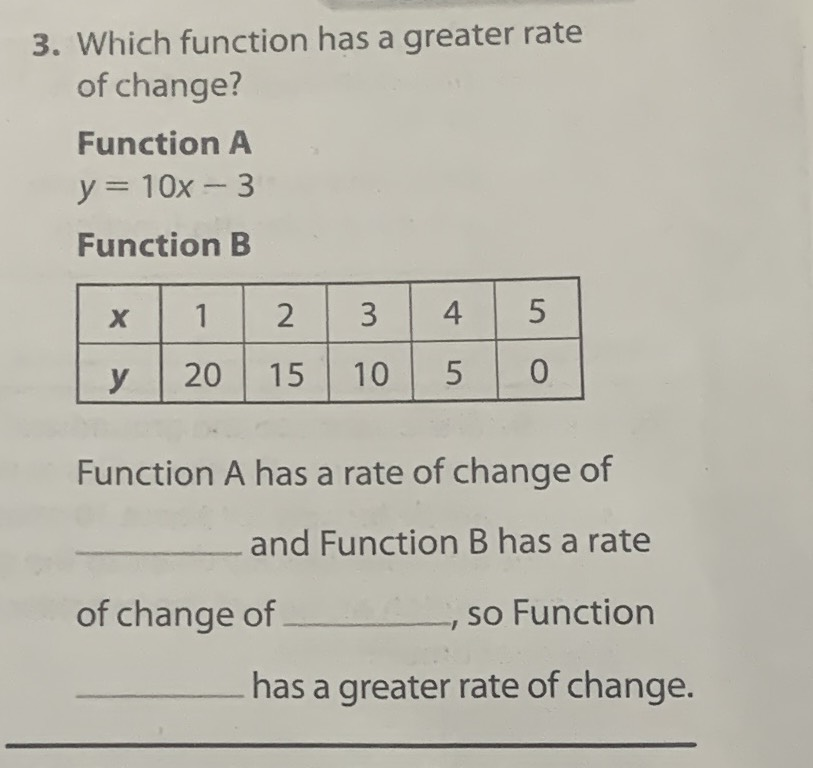### ¿Todavía tienes preguntas de matemáticas?

Pregunte a nuestros tutores expertos
Algebra
Pregunta3. Which function has a greater rate of change?

Function $$A$$

$$y = 10 x - 3$$

Function B

Function $$A$$ has a rate of change of _and Function $$B$$ has a rate of change of _,so Function ._has a greater rate of change.